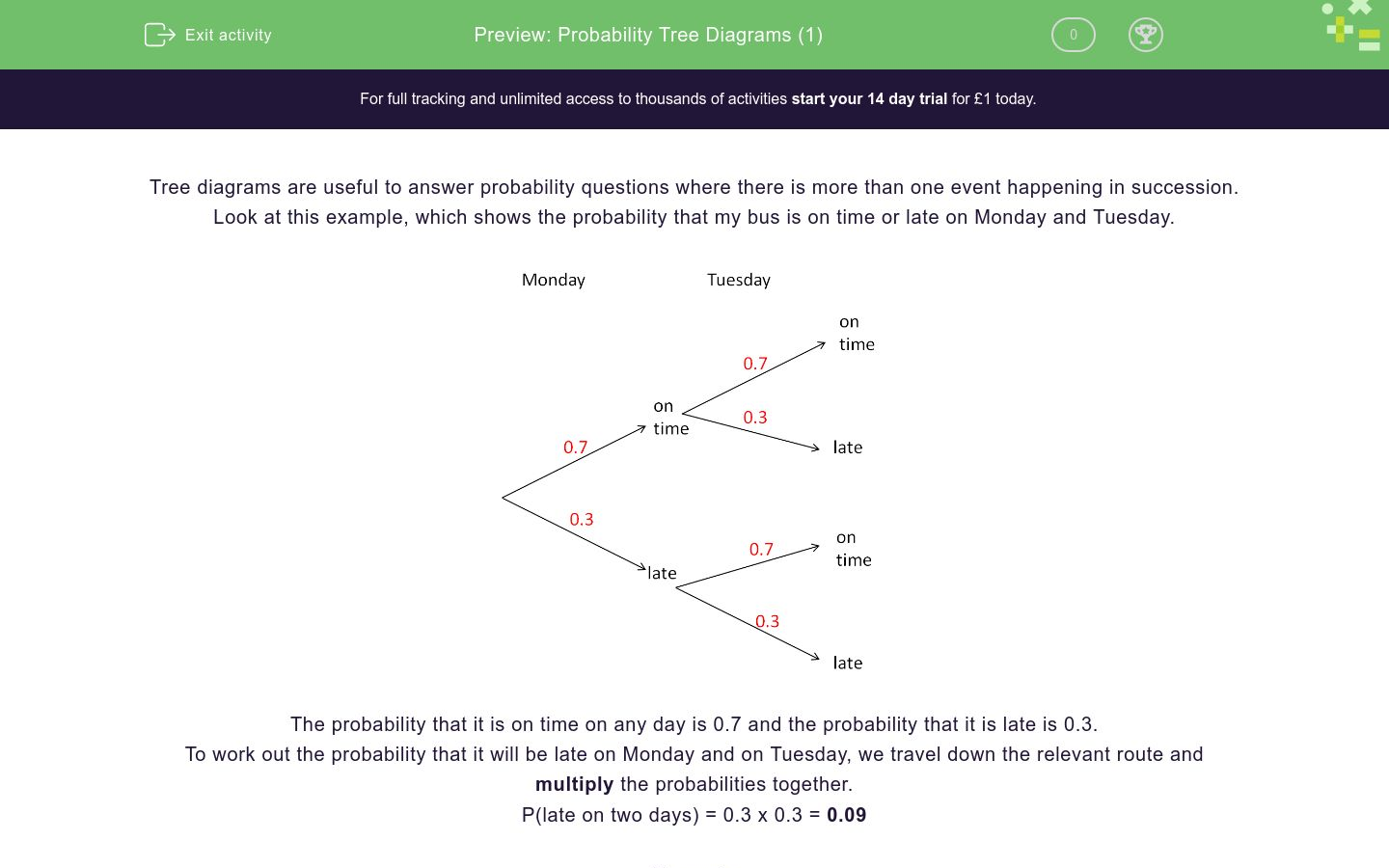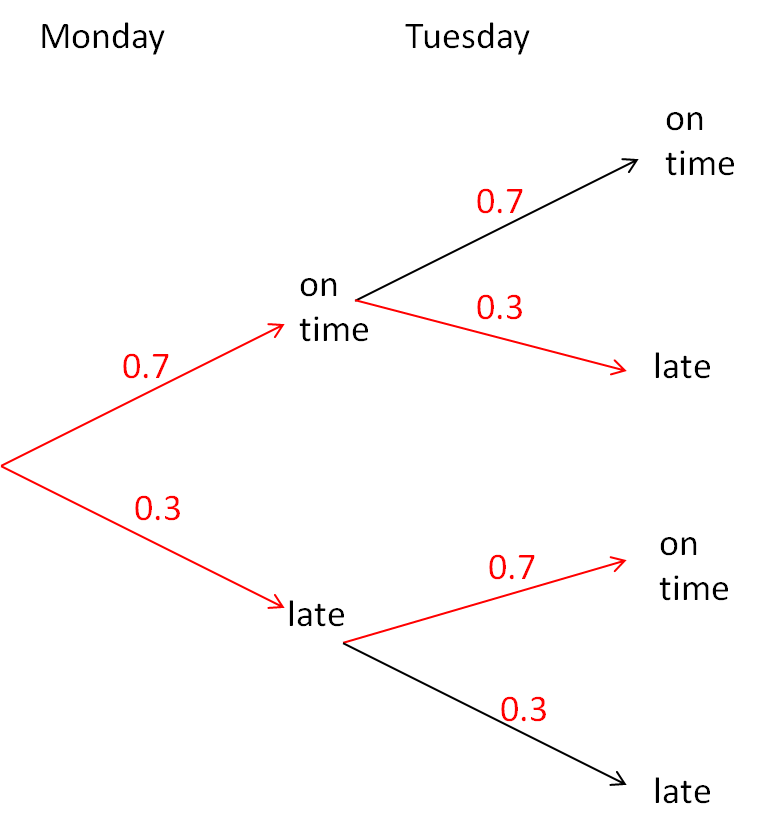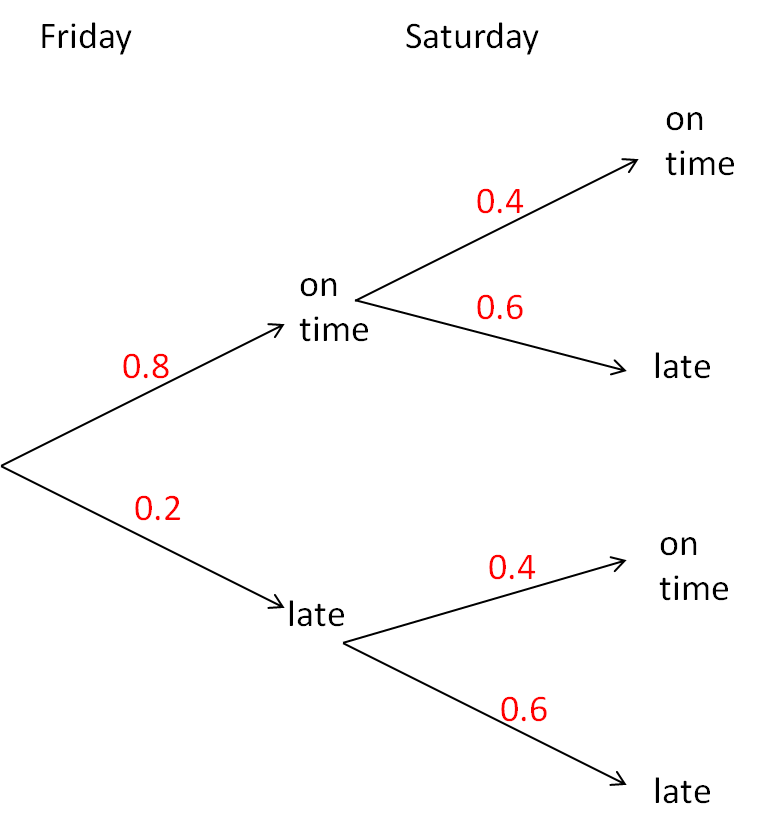# Probability Tree Diagrams (1)

In this worksheet, students understand and use two-stage tree diagrams to answer probability questions.Key stage:  KS 3

Curriculum topic:   Probability

Curriculum subtopic:   Calculate Theoretical Probability

Difficulty level:### QUESTION 1 of 10

Tree diagrams are useful to answer probability questions where there is more than one event happening in succession.

Look at this example, which shows the probability that my bus is on time or late on Monday and Tuesday.The probability that it is on time on any day is 0.7 and the probability that it is late is 0.3.

To work out the probability that it will be late on Monday and on Tuesday, we travel down the relevant route and multiply the probabilities together.

P(late on two days) = 0.3 x 0.3 = 0.09

Example

What is the probability that my bus will be late on only one of the days?

There are two options here, clearly visible on the tree diagram and shown in red below.P(late on Monday only) = 0.3 x 0.7 = 0.21

P(late on Tuesday only) = 0.7 x 0.3 = 0.21

We must now add these two probabilities, because either option is relevant.

P(late on one day only) = 0.21 + 0.21 = 0.42

The tree diagram shows the probabilities that my bus is on time or late on Monday and Tuesday.

Calculate the probability that it will be on time on both days.The tree diagram shows the probabilities that my bus is on time or late on Monday and Tuesday.

Calculate the probability that it will be late on Monday and then on time on Tuesday.The tree diagram shows the probabilities that my bus is on time or late on Wednesday and Thursday.

Calculate the probability that it will be late on Wednesday and then on time on Thursday.The tree diagram shows the probabilities that my bus is on time or late on Wednesday and Thursday.

Calculate the probability that it will be late on both days.The tree diagram shows the probabilities that my bus is on time or late on Wednesday and Thursday.

Calculate the probability that it will be on time on both days.The tree diagram shows the probabilities that my bus is on time or late on Wednesday and Thursday.

Calculate the probability that it will be on time on one of the days and late on the other.The tree diagram shows the probabilities that my bus is on time or late on Friday and Saturday.

Buses are usually late on Saturdays because it is market day.

Calculate the probability that it will be on time on both days.The tree diagram shows the probabilities that my bus is on time or late on Friday and Saturday.

Buses are usually late on Saturdays because it is market day.

Calculate the probability that it will be late on both days.The tree diagram shows the probabilities that my bus is on time or late on Friday and Saturday.

Buses are usually late on Saturdays because it is market day.

Calculate the probability that it will be on time on Friday but late on Saturday.The tree diagram shows the probabilities that my bus is on time or late on Friday and Saturday.

Buses are usually late on Saturdays because it is market day.

Calculate the probability that it will be on time on one day and late on the other.• Question 1

The tree diagram shows the probabilities that my bus is on time or late on Monday and Tuesday.

Calculate the probability that it will be on time on both days.0.49
• Question 2

The tree diagram shows the probabilities that my bus is on time or late on Monday and Tuesday.

Calculate the probability that it will be late on Monday and then on time on Tuesday.0.21
• Question 3

The tree diagram shows the probabilities that my bus is on time or late on Wednesday and Thursday.

Calculate the probability that it will be late on Wednesday and then on time on Thursday.0.24
• Question 4

The tree diagram shows the probabilities that my bus is on time or late on Wednesday and Thursday.

Calculate the probability that it will be late on both days.0.16
• Question 5

The tree diagram shows the probabilities that my bus is on time or late on Wednesday and Thursday.

Calculate the probability that it will be on time on both days.0.36
• Question 6

The tree diagram shows the probabilities that my bus is on time or late on Wednesday and Thursday.

Calculate the probability that it will be on time on one of the days and late on the other.0.48
• Question 7

The tree diagram shows the probabilities that my bus is on time or late on Friday and Saturday.

Buses are usually late on Saturdays because it is market day.

Calculate the probability that it will be on time on both days.0.32
• Question 8

The tree diagram shows the probabilities that my bus is on time or late on Friday and Saturday.

Buses are usually late on Saturdays because it is market day.

Calculate the probability that it will be late on both days.0.12
• Question 9

The tree diagram shows the probabilities that my bus is on time or late on Friday and Saturday.

Buses are usually late on Saturdays because it is market day.

Calculate the probability that it will be on time on Friday but late on Saturday.0.48
• Question 10

The tree diagram shows the probabilities that my bus is on time or late on Friday and Saturday.

Buses are usually late on Saturdays because it is market day.

Calculate the probability that it will be on time on one day and late on the other.0.56
EDDIE SAYS
0.48 + 0.08
---- OR ----

Sign up for a £1 trial so you can track and measure your child's progress on this activity.

### What is EdPlace?

We're your National Curriculum aligned online education content provider helping each child succeed in English, maths and science from year 1 to GCSE. With an EdPlace account you’ll be able to track and measure progress, helping each child achieve their best. We build confidence and attainment by personalising each child’s learning at a level that suits them.

Get started